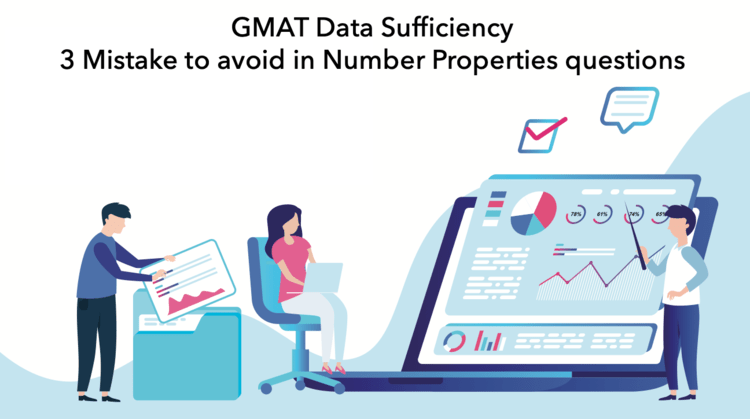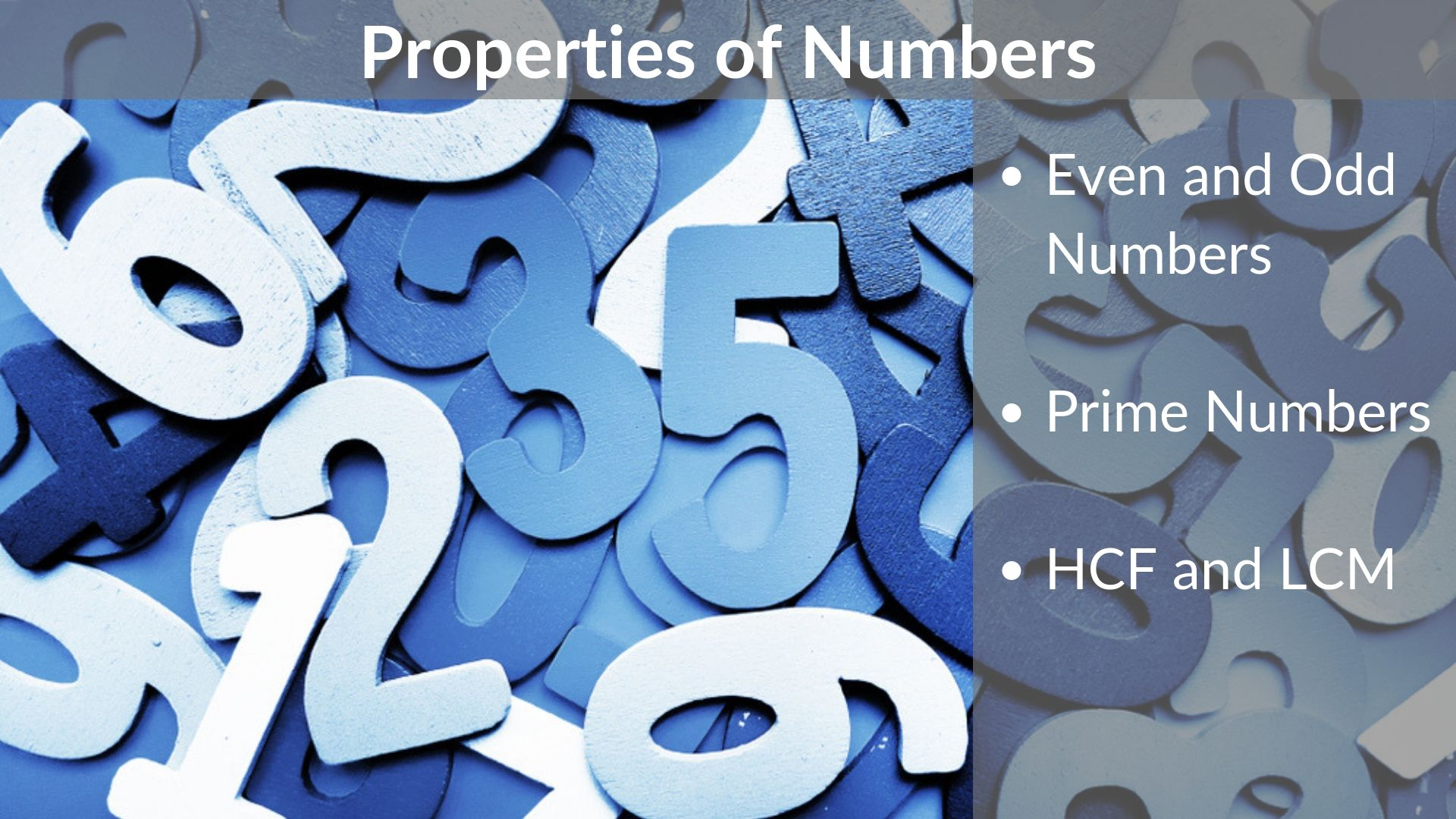# GMAT Data Sufficiency – 3 Mistake Students make in Number Properties questions

Typical GMAT data sufficiency questions are followed by information in the form of two or three statements. You have to figure out which statements you need to correctly answer the question. Many students who do reasonably well in problem-solving questions continue to struggle with GMAT data sufficiency questions. Students often realize that they couldn’t answer

Read More# Properties of Numbers – Even & Odd | Prime | HCF & LCM

Hello Reader, We see that you want to know the basics and properties of numbers. You have landed just at the right place.  Purpose of the article: In this article, You will get a deeper idea about the behavior of numbers and its operations You will also get to know a few facts about the

Read More# GMAT Quant | 3 mistakes in Even-Odd Questions | Number Properties

When it comes to ‘Even – Odd’, it’s not just an issue on Delhi roads, but also in your GMAT Quant section. Even-Odd numbers is deemed to be among the easier concepts on the GMAT Quant, and yet, come 700+ level questions from this concept, many students get them wrong. Our Subject Matter Experts have closely

Read More# Eight light bulbs numbered 1 through 8 are arranged in a circle as shown above. The bulbs are wired so that every third bulb, counting in a clockwise direction, flashes until all bulbs have flashed once. If the bulb numbered 1 flashes first, which numbered bulb will flash last? – OGQR 2020 Question #102 with Solution

Solution Given In this question, we are given Eight light bulbs numbered 1 through 8 are arranged in a circle, as shown in the diagram. The bulbs are wired so that every third bulb, counting in a clockwise direction, flashes until all bulbs have flashed once. The bulb numbered 1 flashes first. To Find We

Read More# If the smaller of 2 consecutive odd integers is a multiple of 5, which of the following could NOT be the sum of these 2 integers? – OGQR 2020 Question #86 with Solution

Solution Given In this question, we are given The smaller of 2 consecutive odd integers is a multiple of 5. To Find We need to determine Among the given options, which one could not be the sum of those 2 consecutive odd integers. Approach & Working Let us assume that the smaller of the 2

Read More# Is the average (arithmetic mean) of the numbers x, y, and z greater than z? – OG 2020 Question #320 with Solution

Solution Steps 1 & 2: Understand Question and Draw Inferences In this question, we are asked to determine whether the average (arithmetic mean) of the numbers x, y, and z is greater than the number z or not. If (x + y +z) / 3  > z Then, x + y + z > 3z

Read More# If x is an integer greater than 0, what is the remainder when x is divided by 4?- OG 2020 Question #318 with Solution

Solution Steps 1 & 2: Understand Question and Draw Inferences In this question, we are given The number x is an integer, and x > 0 We need to determine The remainder, when x is divided by 4. To find the remainder, when x is divided by 4, we need to know either the exact

Read More# If 2.00X and 3.00Y are 2 numbers in decimal form with thousandths digits X and Y, is 3(2.00X) > 2(3.00Y)? – OG 2020 Question #370 with Solution

Solution Steps 1 & 2: Understand Question and Draw Inferences In this question, we are given 2.00X and 3.00Y are two numbers in decimal dorm with thousandths digits X and Y. We need to determine Whether 3(2.00X) > 2(3.00Y) or not. We can simplify the given expression as 3(2.00X) > 2(3.00Y) Or, 3(2 + 0.00X)

Read More# A certain list, L, contains a total of n numbers, not necessarily distinct, that are arranged in increasing order. – OG 2020 Question #377 with Solution

Read MoreRead More